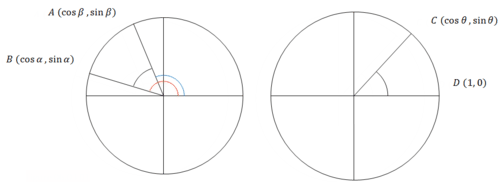# 6.3: Sum and Difference Identities

•• Contributed by CK12
• CK12

With your knowledge of special angles like the sine and cosine of $$30^{\circ}$$ and $$45^{\circ}$$, you can find the sine and cosine of $$15^{\circ}$$, the difference of $$45^{\circ}$$ and $$30^{\circ}$$, and $$75^{\circ}$$, the sum of $$45^{\circ}$$ and $$30^{\circ}$$. Using what
you know about the unit circle and the sum and difference identities, how do you determine $$\sin 15^{\circ}$$ and $$\sin 75^{\circ}$$ ?

### Sum and Difference Identities

First look at the derivation of the cosine difference identity:

$$\cos (\alpha-\beta)=\cos \alpha \cos \beta+\sin \alpha \sin \beta$$Start by drawing two arbitrary angles $$\alpha$$ and $$\beta$$. In the image above $$\alpha$$ is the angle in red and $$\beta$$ is the angle in blue. The difference $$\alpha-\beta$$ is noted in black as $$\theta$$. The reason why there are two pictures is because the image on the right has the same angle $$\theta$$ in a rotated position. This will be useful to work with because the length of $$\overline{A B}$$ will be the same as the length of $$\overline{C D}$$.

\begin{aligned} \overline{A B} &=\overline{C D} \\ \sqrt{(\cos \alpha-\cos \beta)^{2}+(\sin \alpha-\sin \beta)^{2}} &=\sqrt{(\cos \theta-1)^{2}+(\sin \theta-0)^{2}} \\(\cos \alpha-\cos \beta)^{2}+(\sin \alpha-\sin \beta)^{2} &=(\cos \theta-1)^{2}+(\sin \theta-0)^{2} \end{aligned}

$$(\cos \alpha)^{2}-2 \cos \alpha \cos \beta+(\cos \beta)^{2}+(\sin \alpha)^{2}-2 \sin \alpha \sin \beta+(\sin \beta)^{2}=(\cos \theta-1)^{2}+(\sin \theta)^{2}$$

\begin{aligned} 2-2 \cos \alpha \cos \beta-2 \sin \alpha \sin \beta &=(\cos \theta)^{2}-2 \cos \theta+1+(\sin \theta)^{2} \\ 2-2 \cos \alpha \cos \beta-2 \sin \alpha \sin \beta &=1-2 \cos \theta+1 \\-2 \cos \alpha \cos \beta-2 \sin \alpha \sin \beta &=-2 \cos \theta \\ \cos \alpha \cos \beta+\sin \alpha \sin \beta &=\cos \theta \\ &=\cos (\alpha-\beta) \end{aligned}

You can use this identity to prove the cosine of a sum identity. First, start with the cosine of a difference and make a substitution.  Then use the odd-even identity.

$$\cos \alpha \cos \beta+\sin \alpha \sin \beta=\cos (\alpha-\beta)$$

Let $$\gamma=-\beta$$

$$\cos \alpha \cos (-\gamma)+\sin \alpha \sin (-\gamma)=\cos (\alpha+\gamma)$$
$$\cos \alpha \cos \gamma-\sin \alpha \sin \gamma=\cos (\alpha+\gamma)$$

The proofs for sine and tangent are left to the videos and examples.  They are listed here for your reference.  Cotangent, secant and cosecant are excluded because you can use reciprocal identities to get those once you have sine, cosine and tangent.

#### Summary

• $$\cos (\alpha \pm \beta)=\cos \alpha \cos \beta \mp \sin \alpha \sin \beta$$
• $$\sin (\alpha \pm \beta)=\sin \alpha \cos \beta \pm \cos \alpha \sin \beta$$
• $$\tan (\alpha \pm \beta)=\frac{\sin (\alpha \pm \beta)}{\cos (\alpha \pm \beta)}=\frac{\tan \alpha \pm \tan \beta}{1 \mp \tan \alpha \tan \beta}$$

The order of the plus or minus signs is important because for cosine of a sum, the negative sign is used on the other side of the identity.  This is the opposite of sine of a sum, where a positive sign is used on the other side of the identity.

### Examples

Example 1

Earlier, you were asked to evaluate $$\sin 15^{\circ}$$ and $$\sin 75^{\circ}$$ exactly without a calculator. To do this you need to use the sine of a difference and sine of a sum.
$$\begin{array}{l} \sin \left(45^{\circ}-30^{\circ}\right)=\sin 45^{\circ} \cos 30^{\circ}-\cos 45^{\circ} \sin 30^{\circ}=\frac{\sqrt{2}}{2} \cdot \frac{\sqrt{3}}{2}-\frac{\sqrt{2}}{2} \cdot \frac{1}{2}=\frac{\sqrt{6}-\sqrt{2}}{4} \\ \sin \left(45^{\circ}+30^{\circ}\right)=\sin 45^{\circ} \cos 30^{\circ}+\cos 45^{\circ} \sin 30^{\circ}=\frac{\sqrt{2}}{2} \cdot \frac{\sqrt{3}}{2}+\frac{\sqrt{2}}{2} \cdot \frac{1}{2}=\frac{\sqrt{6}+\sqrt{2}}{4} \end{array}$$

Example 2

Find the exact value of $$\tan 15^{\circ}$$ without using a calculator.

$$\tan 15^{\circ}=\tan \left(45^{\circ}-30^{\circ}\right)=\frac{\tan 45^{\circ}-\tan 30^{\circ}}{1+\tan 45^{\circ} \tan 30^{\circ}}=\frac{1-\frac{\sqrt{3}}{3}}{1+1 \cdot \frac{\sqrt{3}}{3}}=\frac{3-\sqrt{3}}{3+\sqrt{3}}$$

A final solution will not have a radical in the denominator.  In this case multiplying through by the conjugate of the denominator will eliminate the radical.

$$=\frac{(3-\sqrt{3}) \cdot(3-\sqrt{3})}{(3+\sqrt{3}) \cdot(3-\sqrt{3})}$$
$$=\frac{(3-\sqrt{3})^{2}}{9-3}$$
$$=\frac{(3-\sqrt{3})^{2}}{6}$$

Example 3

Evaluate the expression exactly without using a calculator.

$$\cos 50^{\circ} \cos 5^{\circ}+\sin 50^{\circ} \sin 5^{\circ}$$

Once you know the general form of the sum and difference identities then you will recognize this as cosine of a difference.

$$\cos 50^{\circ} \cos 5^{\circ}+\sin 50^{\circ} \sin 5^{\circ}=\cos \left(50^{\circ}-5^{\circ}\right)=\cos 45^{\circ}=\frac{\sqrt{2}}{2}$$

Example 4

Use a sum or difference identity to find an exact value of $$\cot \left(\frac{5 \pi}{12}\right)$$.

\begin{aligned} \cot \left(\frac{5 \pi}{12}\right) &=\frac{1}{\tan \left(\frac{5 \pi}{12}\right)} \\ &=\frac{1}{\tan \left(\frac{9 \pi}{12}-\frac{4 \pi}{12}\right)} \\ &=\frac{1}{\tan \left(135^{\circ}-60^{\circ}\right)} \\ &=\frac{1+\tan 135^{\circ} \tan 60^{\circ}}{\tan 135^{\circ}-\tan 60^{\circ}} \\ &=\frac{1+(-1) \cdot \sqrt{3}}{(-1)-\sqrt{3}} \\ &=\frac{(1-\sqrt{3})}{(-1-\sqrt{3})} \\ &=\frac{(1-\sqrt{3})^{2}}{(-1+\sqrt{3}) \cdot(1-\sqrt{3})} \\ &=\frac{(1-\sqrt{3})^{2}}{-(1-3)} \\ &=\frac{(1-\sqrt{3})^{2}}{2} \end{aligned}

Example 5

Prove the following identity:

$$\frac{\sin (x-y)}{\sin (x+y)}=\frac{\tan x-\tan y}{\tan x+\tan y}$$

Here are the steps:

\begin{aligned} \frac{\sin (x-y)}{\sin (x+y)} &=\frac{\tan x-\tan y}{\tan x+\tan y} \\ \frac{\sin x \cos y-\cos x \sin y}{\sin x \cos y+\cos x \sin y} &=\\ \frac{\sin x \cos y-\cos x \sin y}{\sin x \cos y+\cos x \sin y} \cdot \frac{\left(\frac{1}{\cos x \cdot \cos y}\right)}{\left(\frac{1}{\cos x \cdot \cos y}\right)} &=\\ \frac{\left(\frac{\sin x}{\cos x \cdot \cos y}\right)-\left(\frac{\cos x \sin y}{\cos x \cdot \cos y}\right)}{\left(\frac{\sin x \cos x}{\cos x \cdot \cos y}\right)+\left(\frac{\cos x}{\cos x \cdot \cos y}\right)} &=\\ \frac{\tan x-\tan y}{\tan x+\tan y} &=\end{aligned}

Review

Find the exact value for each expression by using a sum or difference identity.

1. $$\cos 75^{\circ}$$

2. $$\cos 105^{\circ}$$

3. $$\cos 165^{\circ}$$

4. $$\sin 105^{\circ}$$

5. $$\sec 105^{\circ}$$

6. $$\tan 75^{\circ}$$

7. Prove the sine of a sum identity.

8. Prove the tangent of a sum identity.

9. Prove the tangent of a difference identity.

10. Evaluate without a calculator: $$\cos 50^{\circ} \cos 10^{\circ}-\sin 50^{\circ} \sin 10^{\circ}$$.

11. Evaluate without a calculator: $$\sin 35^{\circ} \cos 5^{\circ}-\cos 35^{\circ} \sin 5^{\circ}$$.

12. Evaluate without a calculator: $$\sin 55^{\circ} \cos 5^{\circ}+\cos 55^{\circ} \sin 5^{\circ}$$.

13. If $$\cos \alpha \cos \beta=\sin \alpha \sin \beta,$$ then what does $$\cos (\alpha+\beta)$$ equal?

14. Prove that $$\tan \left(x+\frac{\pi}{4}\right)=\frac{1+\tan x}{1-\tan x}$$

15. Prove that $$\sin (x+\pi)=-\sin x$$.